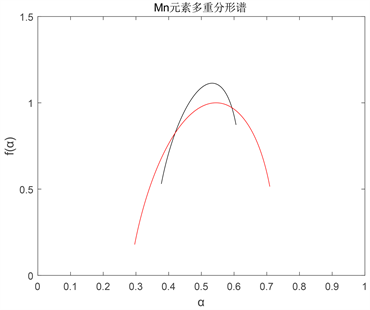# Mn元素多重分形分析Multifractal Analysis of Mn Element

DOI: 10.12677/AAM.2020.94067, PDF, HTML, XML, 下载: 103  浏览: 179  国家自然科学基金支持

Abstract: The study of the distribution law of geochemical elements is one of the important ways to reveal the law of element mineralization and spatial change. Taking the desert region of Yashan, Xinjiang as an example, two types of minerals are selected, combined with multiple fractals, and multiple fractal moment estimation methods are used to conduct a full analysis of the elements in the soil in the two desert regions. From the aspects of singularity and asymmetric index, the non-elements of the elements are further explored. Linear migration provides a new method and direction for prospecting in the desert areas in the future. From the results, we can see that the distribution of the ore-forming element Mn in the soils of regions I and II has continuous multifractal characteris-tics. Then, by comparing the singular and asymmetric indices of the two regions, we find that the singular and asymmetric indices for the values of area I are larger than area II. It can be inferred that the migration characteristics of area I are higher than area II. Therefore, the multifractal characteristics of the elements have certain significance for ore prospecting in desert areas.

1. 引言

2. 多重分形谱分析

$\tau \left(q\right)=\underset{r\to 0}{\mathrm{lim}}\frac{\mathrm{ln}{\chi }_{q}\left(r\right)}{\mathrm{ln}r}$ (1)

$\alpha \left(q\right)=\frac{\partial \left(\tau \left(q\right)\right)}{\partial q}$ (2)

$f\left(\alpha \right)=\alpha \left(q\right)q-\tau \left(q\right)$ (3)

$R=\frac{{\alpha }_{0}-{\alpha }_{\mathrm{min}}-\left({\alpha }_{\mathrm{max}}-{\alpha }_{0}\right)}{\Delta \alpha }$ (4)

3. 数据来源

4. Mn元素全量分析Figure 1. Total multifractal spectrum of Mn (red I, black II)

Mn元素多重分形谱 $f\left(\alpha \right)$ 均表现为钩状且呈连续分布，表明连续多重分形是金属地球化学场元素的一种普遍规律；同时，曲线 $\alpha -f\left(\alpha \right)$ 均为不对称的上凸曲线，表明元素均经历过不同程度的叠加改造，且元素具备多重分形特征 。

Mn元素多重分形数据结果如表1所示， $\Delta \alpha$ 代表谱开口宽度， $\Delta f$ 反映的是左右谱高差，R为非对称指数。从数学方面来分析， $\Delta f$ 显示元素高值区域和低值区域分布的比例， $\Delta f$ 越小，高值区域所占比例越大，分形谱函数 $f\left(\alpha \right)$ 曲线的左半段主要反映了 $q\ge 0$ 的特征，能刻划分形的基本特征，而右半段为 $q<0$ 部分主要反映了测量过程的各种误差或者干扰以及分形结构中的细小结构变化，也就是说低含量的特征能得到加强。开口宽度 $\Delta \alpha$ 反映了元素分布在整个测度上分布的均匀性和奇异性， $\Delta \alpha$ 越大，说明元素在空间上的分布越不均匀。非对称指数R > 0且R值越大，不对称性越明显。从元素迁移方面来说， $\Delta f$ 越小，高值区域所占比例越大，在土壤中的活动性就越强，当地质系统受到微小扰动的时候，元素发生迁移的可能性就越大。 $\Delta \alpha$ 反映了元素分布在整个测度上分布的均匀性和奇异性， $\Delta \alpha$ 越大，奇异性越大，属于越有利迁移的类型。非对称指数R > 0且R值越大，迁移特征越明显，R值的大小与主要成矿元素迁移潜力有一定的对应关系 。Table 1. Mn element multifractal result data

5. 结论

  於崇文. 成矿动力系统在混沌边缘的分形生长——一种新的成矿理论与方法论[J]. 矿物岩石地球化学通报, 2002, 21(2): 103-113.  谢淑云, 焦杨, 燕敏, 信栋林, 徐德义, 成秋明. 白音诺尔矿区土壤地球化学纵向非主要成矿特征[J]. 地球科学(中国地质大学学报), 2012, 37(6): 1140-1148.  申维. 分形理论与矿产预测[M]. 北京: 地质出版社, 2002.  成秋明. 成矿过程奇异性与矿产预测定量化的新理论与新方法[J]. 地学前缘, 2007, 14(5): 42-53.  肖凡, 陈建国, 侯卫生, 王正海. 钦-杭结合带南段庞西垌地区Ag-Au致矿地球化学异常信息识别与提取[J]. 岩石学报, 2017, 33(3): 779-790.  成秋明. 非线性矿床模型与非常规矿产资源评价[J]. 地球科学, 2003, 28(4): 445-454.  成秋明. 非线性成矿预测理论: 多重分形奇异性-广义自相似性-分形谱系模型与方法[J]. 地球科学, 2006, 31(3): 337-348.  李伟, 何福国, 丁汝福, 李昌明, 游军, 邵帅. 内蒙古太平川钼铜矿床蚀变、地球化学异常特征及其找矿意义[J]. 矿产与地质, 2016, 30(6): 887-893.  李晓晖, 袁峰, 周涛发, 邓宇峰, 张达玉, 许超, 张若飞. 新疆塔尔巴哈台-萨吾尔地区多重分形地球化学异常提取及成矿预测[J]. 岩石学报, 2015, 31(2): 426-434.  刘舒飞. 广西右江盆地金异常分布分形特征与成矿预测[J]. 中国矿业, 2018, 27(S1): 143-146.  周杰, 袁峰, 李晓晖, 张明明, 胡训宇, 丁文祥, 胡迪, 李现锁. 皖东张八岭-管店地区Au地球化学异常信息识别与提取[J]. 矿物岩石地球化学通报, 2019, 38(1): 150-158.  Manderbrot, D.B. (1982) The Fractal Geometry of Nature. Freeman and Company, San Francisco, 1-20.  Manderbrot, D.B. (1989) Multifractal Measures, Especially for the Geophysicist. Pure and Applied Geophysics, 131, 5-42. https://doi.org/10.1007/BF00874478  Cheng, Q.M. (1999) Gliding Box Method and Multifractal Modelling. Computer & Geosciences, 25, 1073-1080. https://doi.org/10.1016/S0098-3004(99)00068-0  Cheng, Q.M. (1999) Markov Processes and Discrete Multifractals. Mathematical Geology, 31, 455-469. https://doi.org/10.1023/A:1007594709250  San, J.M., Martín, M.A. and Caniego, F.J. (2010) Multifractal Analysis of Discretized X-Ray CT Images for the Characterization of Soil Macropore Structures. Geoderma, 156, 32-42. https://doi.org/10.1016/j.geoderma.2010.01.004  Cheng, Q.M. (1999) Multifractality and Spatial Statistics. Computers and Geosciences, 25, 949-962. https://doi.org/10.1016/S0098-3004(99)00060-6  Tarquis, A.M. (2009) Pore Network Complexity and Thresholding of 3D Soil Images. Ecological Complexity, 6, 230-239. https://doi.org/10.1016/j.ecocom.2009.05.010  Cheng, Q.M. and Agterberg, F.P. (1995) Multifractal Modeling and Spatial Point Processes. Mathematical Geology, 27, 831-845. https://doi.org/10.1007/BF02087098  Xie, S.Y., Cheng, Q.M., Zhang, S.Y. and Huang, K. (2010) Assessing Microstructures of Pyrrhotites in Basalts by Multifractal Analysis. Nonlinear Processes in Geophysics, 17, 319-327. https://doi.org/10.5194/npg-17-319-2010  谢淑云, 鲍征宇. 多重分形方法在金属成矿潜力评价中的应用[J]. 成都理工大学学报(自然科学版), 2004, 31(1): 28-33.